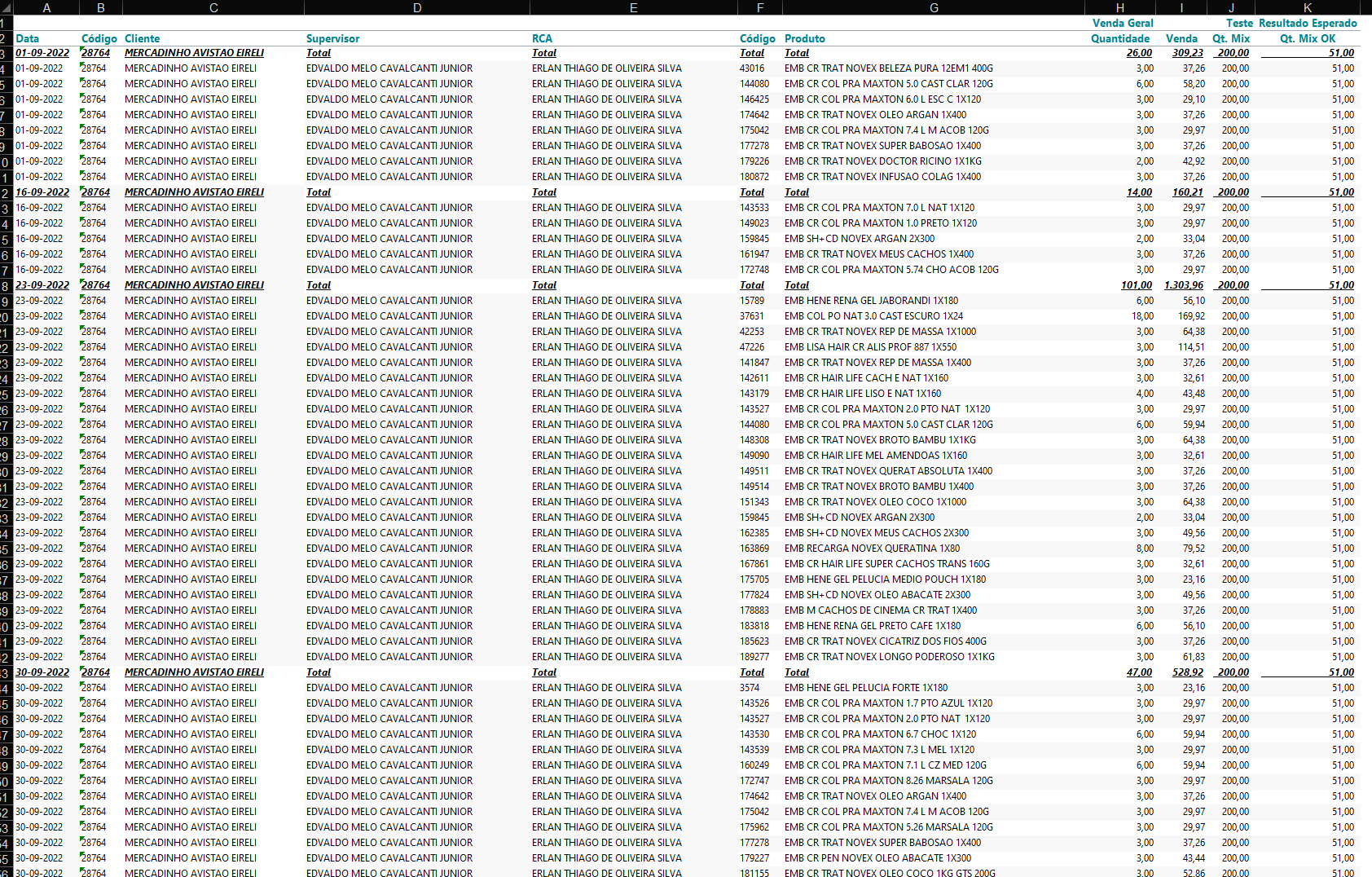# Calculation to find the mix totals and subtotals by customers for a given period

Hello everybody,

I have the following table in TARGIT, but I need to perform the calculation on a column that
I described it as "Expected Result", where in the test column it didn't work.

What I want is, to count and replicate for each line, the total of the mix obtained by the client, regardless of the date. That is, selecting the period that the table shows to be two months, calculate the total of the mix. And behave towards other customers.• Hi Willer,

If I understand you correct, the "200" is wrong and the "51" is correct.

Can you explain a bit more on how you get to the 51?

BR / Ole

• Hello Ole Dyring
So, 51 would be the information I want to get, where 51 is the number of positive items in general, and I want this result to be replicated line by line. As shown in the example table. This is the result that the client performed in these periods shown in the table.